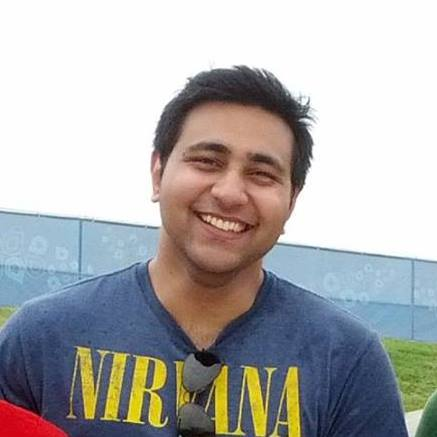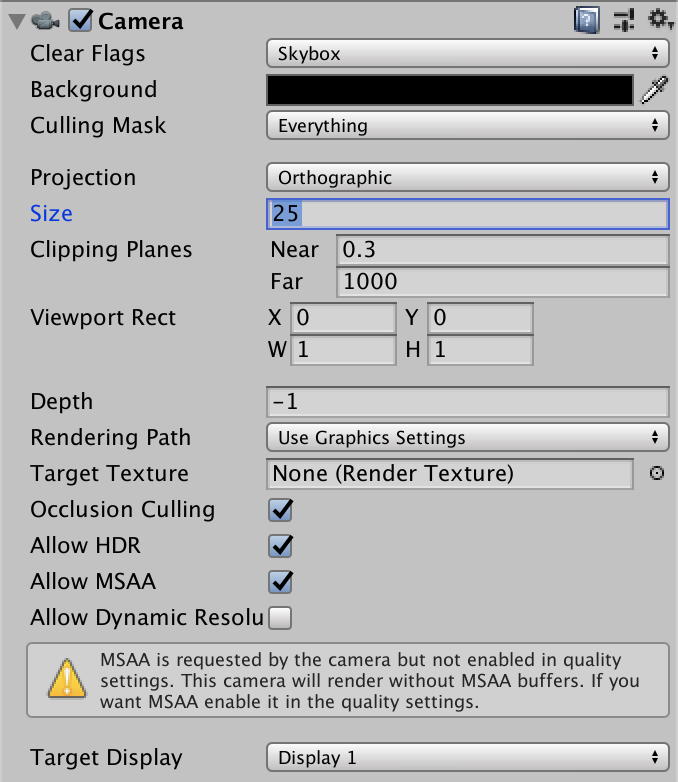## Pronoy Chopra

Developer/Engineer

Theme: plainwhite

## Unity2D - Orthographic camera size

I’ve decided to learn Unity2D by building old games. I started off by building Pong from this tutorial. Obviously, learned a lot.

I wanted to tackle Snake on my own but I got stuck (for days) on how to place the borders on a free aspect camera. First things first - what is the relation between Unity units and pixels. Well, you can decide what it means for every sprite. In this case, it was one pixel per unit for the border sprites.In the above image the camera size is 25, which means it’s 50 units in height. However the width is determined by the aspect ratio of the screen. Since you don’t want to restrict the screen size (or at least you shouldn’t), you have to calculate the relative coordinates to place your assets.

Here’s how I did that (I only did borders right and left because vertical camera size doesn’t change)

``````// calculate height
float height = 2f * m_OrthographicCamera.orthographicSize;
// calculate width
float width = height * m_OrthographicCamera.aspect;

// my asset is 1px wide, so from the center of the asset it's 0.5f to either edge of the asset
// which means you want to position it at the edge of the width - 0.5f
float leftBorderPosition = -1f * (width / 2) + 0.5f;
// same with the right border
float rightBorderPosition = width / 2 - 0.5f;

// position your assets with a new vector3
BorderLeft.transform.position = new Vector3(leftBorderPosition, 0, 0);
BorderRight.transform.position = new Vector3(rightBorderPosition, 0, 0);

// we want to also scale our border asset to the entire width
// so we should calculate the ratio for the x aspect
// grab the size of the asset
float spriteWidth = BorderTop.GetComponent<SpriteRenderer>().bounds.size.x;
// grab the ratio for scale
float ratio = width / spriteWidth;

// and apply it
BorderTop.transform.localScale = new Vector3(ratio, 1, 1);
BorderBottom.transform.localScale = new Vector3(ratio, 1, 1);
``````

This way, assets can be placed relative to the aspect ratio when the game is first booted up.